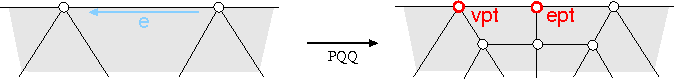## CGAL::CatmullClark_mask_3<Polyhedron_3>

### Definition

A stencil determines a source neighborhood whose points contribute to the position of a refined point. The geometry mask of a stencil specifies the computation on the nodes of the stencil. CatmullClark_mask_3<Polyhedron_3> implements the geometry masks of Catmull-Clark subdivision on a Polyhedron_3<Cartesian>.

#include <CGAL/Subdivision_mask_3.h>

### Parameters

The full template declaration of CatmullClark_mask_3<Polyhedron_3> states one template parameter:

 template < class Polyhedron_3> class CatmullClark_mask_3;

The only parameter requires a Polyhedron_3 as the argument. The Polyhedron_3 should be specialized with the Cartesian kernel, which defines the Point_3 for the vertices.

### Creation

 CatmullClark_mask_3 CC; default constructor.

### Stencil functions

 void CC.facet_node ( Facet_handle f, Point_3& pt) computes the Catmull-Clark facet-point pt of the facet f. void CC.edge_node ( Edge_handle e, Point_3& pt) computes the Catmull-Clark edge-point pt of the edge e. void CC.vertex_node ( Vertex_handle v, Point_3& pt) computes the Catmull-Clark vertex-point pt of the vertex v. void CC.border_node ( Halfedge_handle e, Point_3& ept, Point_3& vpt) computes the Catmull-Clark edge-point ept and the Catmull-Clark vertex-point vpt of the border edge e.